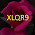## Sunday, November 23, 2014

### Solving Problems on the Concept of P N Junction Diode

PN junction diode is a electronic device which acts like a current passing device in forward bias and acts like a insulator when it is in the reverse bias. We had already analyzed the working the of the PN junction diode in the previous post.Here we would like to analyse some more problems on this concept.The core point that we have to understand is when the voltage applied to the P type material is more than that of the N type than the diode is in the forward bias and it is able to pass the current through it .

Let us consider solving the problem on the concept of a PN junction now.In the following problem we have to identify which of the diode is in forward bias in which of the diode is in reverse Bias.

In solving the problem we have to remember only one simple concept that if the voltage that is applied to the P type material of the PN junction diode is higher than that of the voltage that is applied to the N type material of the PN junction diode,than the diode is said to be in the forward bias and vice versa.

Let us solve one more problem on the same concept. In this problem calculate the current passing in the circuit.

While we are solving this problem we have to understand that the diode is in the forward bias and it can be able to pass the current through it. In the case of an ideal diode,the resistance of the diode in the forward bias is equal to zero.When no information is given in the problem the shall assume that diode is ideal .

Problem and Solution

Let us see one more problem in solving.In this problem we have to find the current passing through the hundred ohm resistor.

After having a look at the circuit we can understand that the diode two is in the reverse Bias and there is no way that the current is going to passing that diode. Thus that part of the circuit will become the dead part of the circuit and it is not going to pass any part of the current through it.

Here we would like to solve one more problem basing on the diodes and their way of connection. Find the equivalent resistance between the point A and B  as shown in the following circuit.

We need to solve the problem in the two following conditions.

What happens the effective resistance when the voltage at the point A  is greater than voltage at the point B and what happens to effective resistance when the voltage at the point B is greater than that of the voltage at the pointy A ?

In solving the problem we are to be little bit more careful. First of all we had understand that the if diode is in the forward bias it will pass the current through it with the zero  resistance.When the diode is the reverse Bias it will not pass the current through it.

When the voltage at the pointy A is greater than that of the voltage at the point B the current the directed from the pointy A , has two options either  to go through the 18 ohms are to pass to the diode D1. The entire current choose to pass through the diode D1 because the diode D1 is not offering any resistance and simply because it is in the forward bias. Current always prefer pass into the devise ways having less resistance and it will never pass through the diode who is having high resistance.
when there is an option of having a devise with zero resistance. Hence the current the distracted from the pointy a will pass through the diode D1 and then through the diode D2 and then to the diode D3 and finally reach the point be bypassing all the available resistances.

Hence the total resistance is simply sum of all these resistance because they are all in series.The same kind of analysis can be made even with the second case also.

Problem and Solution

Find the current passing through the battery in the following circuit.
Here also we are going to follow the same technique.Tthe diode is in forward bias will pass the current in the diode who is in reverse bias,it will not pass the current is the simple concept that we need to know to solve this problem.

Problem and Solution

Find the equal and resistance between the point A and B  when the voltage applied  at the pointy A is greater than that of the voltage at the point B.

The analysis of the problem with is done with the blue colour ink as shown in the diagram below. We had analyse the problem bit more carefully while we are solving.

What all explanation  being written in the diagram itself and we want you to have a look at that and ask the questions that if you have, you can just commented the end of the page so that , we will try to clarify.

Related Post

1.good collection of conceptual problems sir

2.Very clear explanation.Really helpful for beginners.

3.good explanation

4.Sir thank u for clearly explainimg